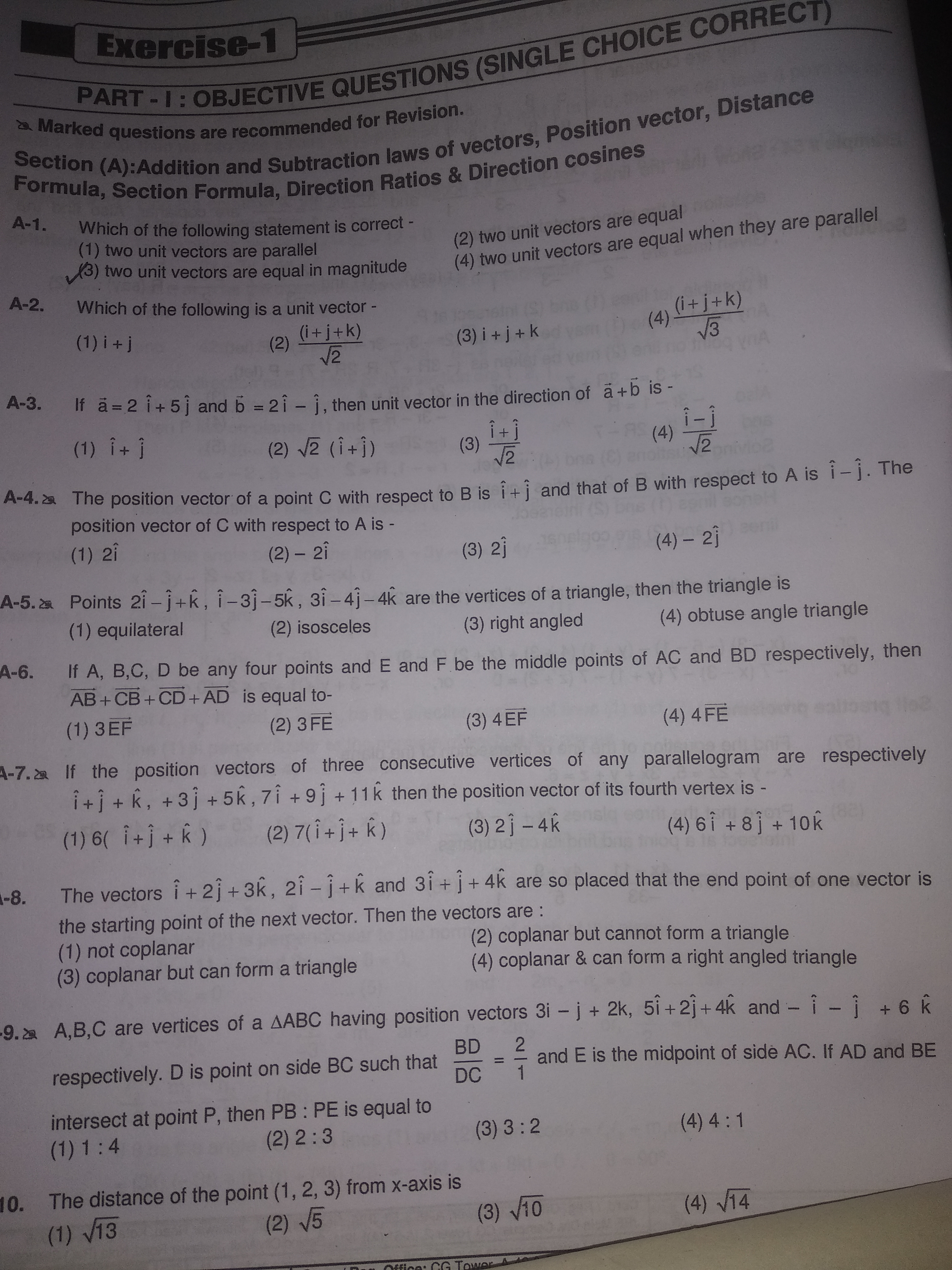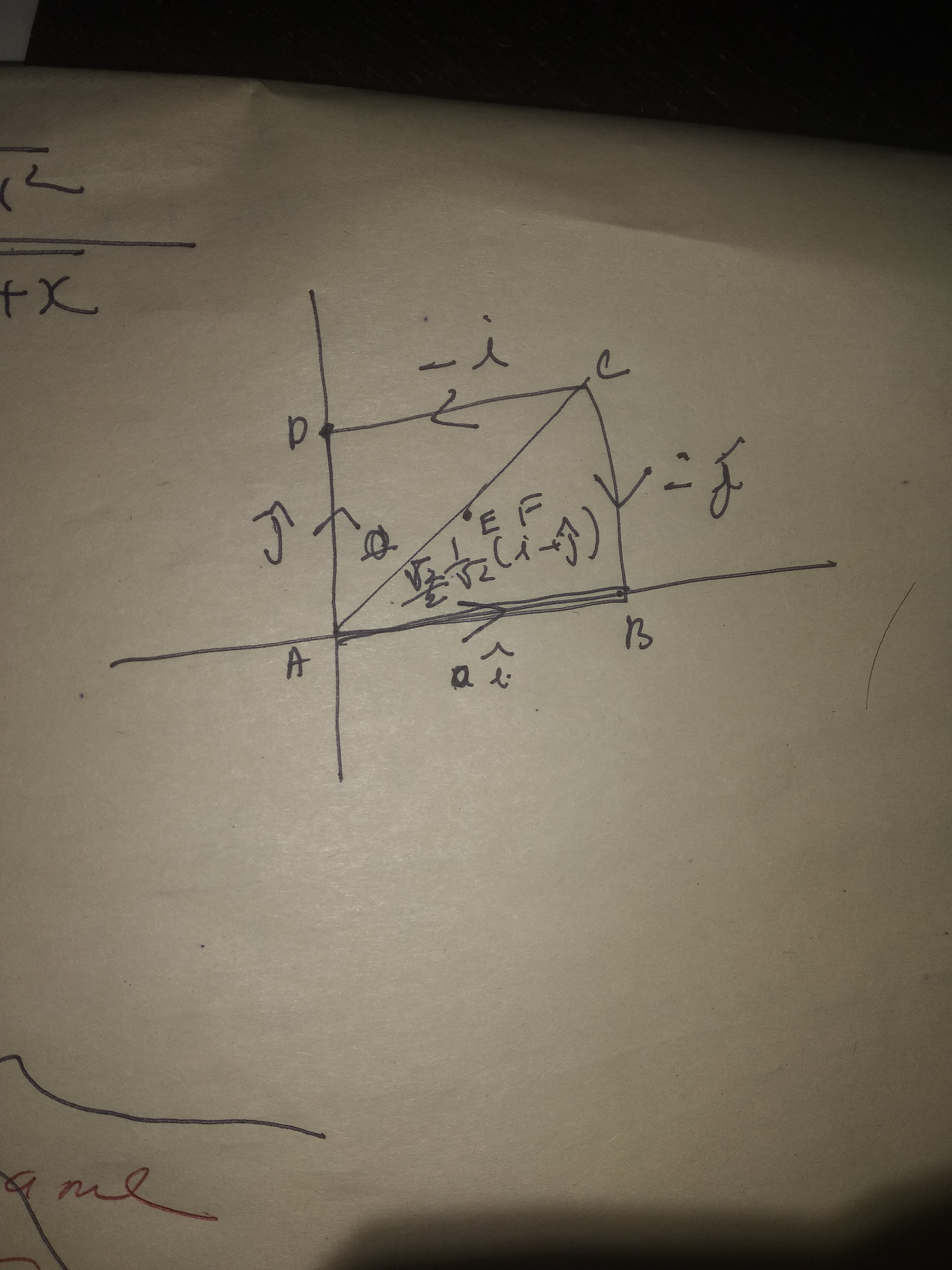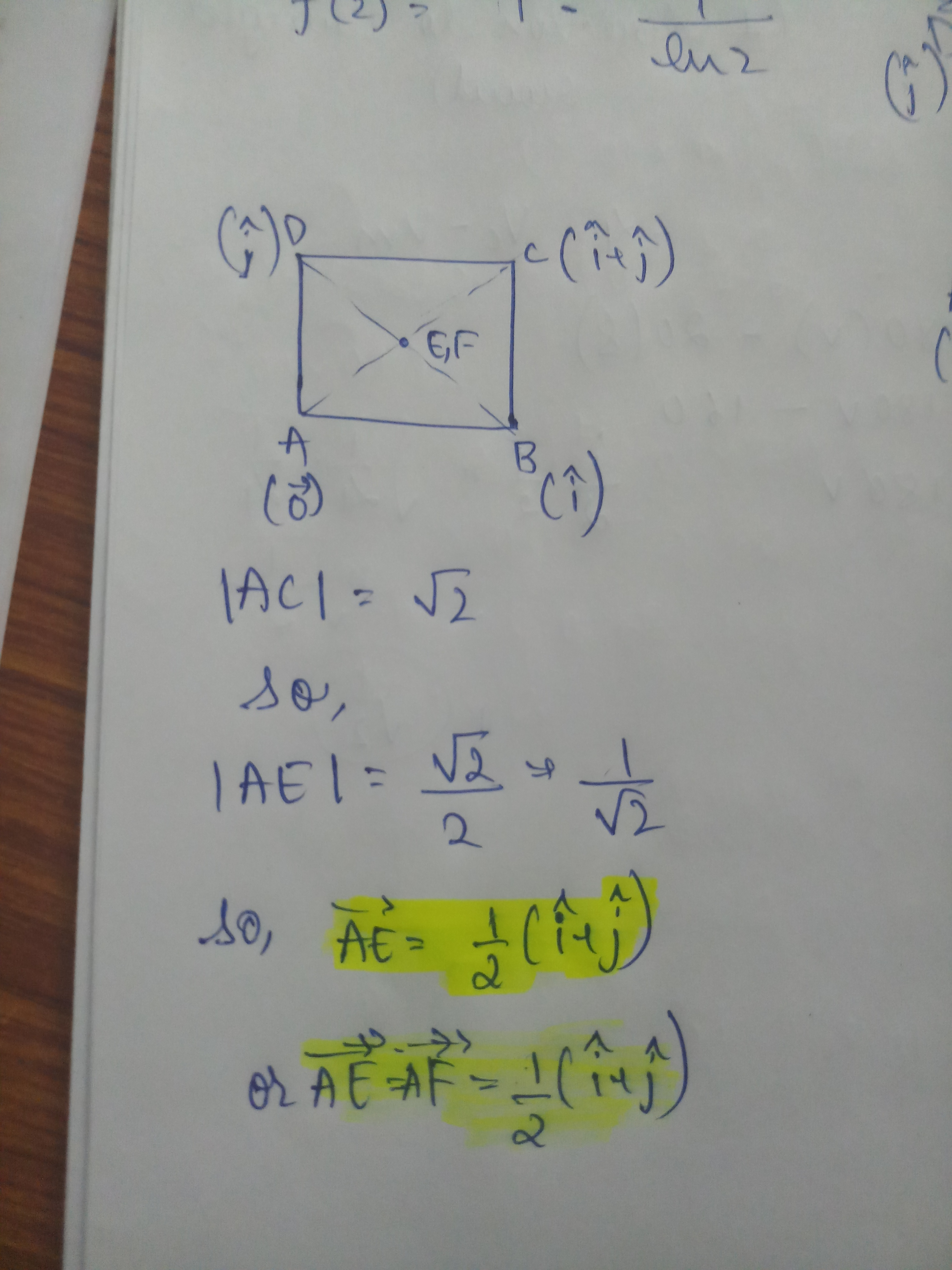# Doubt from vectorsA6
As they have said any four points so I have taken a square but than the resultant sum is coming zero which is matching with no option, what is the mistake?Here as you calculate the vector sum to be 0 it is satisfying all options as EF vector = 0 vector.

You have taken special case and hence you this assumption will mot eliminate any options as all the 4 options are satisfying itSince E F vectors are same in square, u can try another quadrilateral